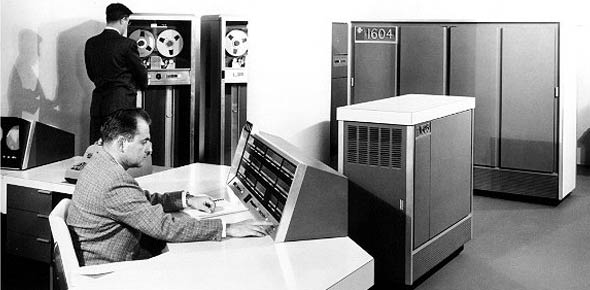# CDC 3D153 Volume 2 V2 (April 2013)

100 Questions | Total Attempts: 1979SettingsCDC 3D153 01 1206, Edit Code 02 (April 2013) Volume 2 Units 1-5 Unit 1: Antenna Theory Unit 2: Electromagnetic Wave Theory Unit 3: Transmission Security Techniques, Data Linking Concepts and Jam-Resistant Communications Unit 4: Other RF Transmission Systems Unit 5: High Frequency Communications

• 1.
Equipment used for generating, amplifying, and transmitting RF carrier is collectively called
• A.

• B.

An antenna.

• C.

A transmitter.

• D.

A transmission line.

• 2.
What basic receiver function involves having the transmitted electromagnetic wave pass through the receive antenna in such a manner as to induce a voltage in the antenna?
• A.

Reproduction.

• B.

Reception.

• C.

Detection.

• D.

Selection.

• 3.
The ability of a receiver to reproduce the signal of a very weak station is the characteristic of the receiver’s
• A.

Fidelity.

• B.

Capacity.

• C.

Sensitivity.

• D.

Selectivity.

• 4.
The ability to reproduce the input signal accurately is the characteristics of the receiver’s
• A.

Fidelity.

• B.

Capacity.

• C.

Sensitivity.

• D.

Selectivity.

• 5.
Which of the following CANNOT be performed by the transceiver’s computerized components and micro-circuitry?
• A.

Specific equipment faults.

• B.

Internal equipment tests.

• C.

Internal calibrations.

• D.

Repair itself.

• 6.
Resistance of the conductor material in a transmission line leads to which type of loss?
• A.

Copper.

• B.

• C.

Inductive.

• D.

Skin-effect.

• 7.
Leakage loss in a transmission line can be minimized by using a
• A.

Thinner conductor.

• B.

Thicker conductor.

• C.

Very low-resistance dielectric.

• D.

Very high-resistance dielectric.

• 8.
A transmission line that consists of a center conductor placed inside a rigid metal tube functioning as the outer tube is called a
• A.

Flexible coaxial cable.

• B.

Rigid coaxial cable.

• C.

Waveguide.

• D.

• 9.
Which statement concerning waveguides is FALSE?
• A.

Very slight damage to the external surface of a waveguide can cause arcing.

• B.

Their conductive material construction is easily dented.

• C.

Effectiveness of waveguides is hindered by moisture.

• D.

Waveguides can be destroyed by corrosion.

• 10.
What two properties of a transmission line determine its characteristic impedance?
• A.

Inductance and resistance.

• B.

Inductance and capacitance.

• C.

Resistance and capacitance.

• D.

Length and type of shielding.

• 11.
Using figure 1–10, determine the electrical length if the frequency is changed from 150 MHz to 250 MHz.
• A.

8.33 meters.

• B.

8.33 wavelengths.

• C.

83.3 meters.

• D.

83.3 wavelengths.

• 12.
Which statement concerning line lengths is TRUE?
• A.

Frequency and electrical length are inversely proportional.

• B.

Frequency and physical length are directly proportional.

• C.

With constant physical length, frequency and electrical length are directly related.

• D.

With constant physical length, frequency and electrical length are inversely related.

• 13.
A nonresonant transmission line is a line
• A.

Having reflected waves.

• B.

Having no reflected waves.

• C.

With maximum voltage across its open termination.

• D.

With maximum voltage across its shorted termination.

• 14.
If a transmission line is terminated in an open, what will likely result?
• A.

Signal loss would be negligible.

• B.

There would be significant signal loss.

• C.

Current would be at maximum at the termination.

• D.

Voltage would be at minimum at the termination.

• 15.
The concept that alternating current changes in magnitude and reverses its direction during each cycle is
• A.

An unproven hypothesis.

• B.

The definition of propagation.

• C.

• D.

What led to the discovery of direct current.

• 16.
At what point do magnetic fields around a wire no longer have time to collapse completely between alternations?
• A.

60 cycles per second (cps).

• B.

120 cps.

• C.

10,000 cps.

• D.

15,000 cps.

• 17.
Which basic field detaches from the antenna and travels through space at great distances?
• A.

• B.

Induction.

• C.

Gravitational.

• D.

Electromotive.

• 18.
Areas in which no antenna radiation pattern passes through are called
• A.

Nulls.

• B.

Lobes.

• C.

Free space.

• D.

Nonresonant.

• 19.
If the electric field component travels in a plane perpendicular to the Earth’s surface, the radio wave is considered to be polarized
• A.

Magnetically.

• B.

Horizontally.

• C.

Circularly.

• D.

Vertically.

• 20.
The actual range of frequencies within the antenna’s bandwidth is called the
• A.

Wavelength.

• B.

Operating zone.

• C.

Frequency range.

• D.

Bandwidth range.

• 21.
What measurement is used to determine whether an antenna is resonant at a particular frequency?
• A.

Distortion.

• B.

Standing wave ratio.

• C.

Signal-to-noise ratio.

• D.

Percent of modulation.

• 22.
The ability of an antenna to both receive and transmit equally well is known as the antenna’s
• A.

Bandwidth.

• B.

Resonance.

• C.

Reciprocity.

• D.

Effectiveness.

• 23.
The standard used to measure the radiating effectiveness (gain) of an antenna system is the
• A.

Marconi antenna.

• B.

Isotropic antenna.

• C.

Hertz antenna.

• D.

Whip antenna.

• 24.
What does the overall effectiveness of an entire transmitting and receiving system depend largely upon?
• A.

Impedance matching.

• B.

Antenna distance.

• C.

Antenna properties.

• D.

Line characteristics.

• 25.
• A.

Omni-directional.

• B.

Uni-directional.

• C.

Bi-directional.

• D.

Directional.

Related TopicsBack to top